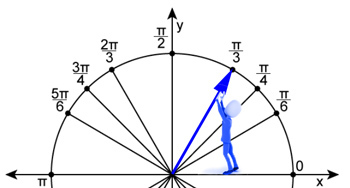• ### Browse All Lessons

##### Assign Lesson

Help Teaching subscribers can assign lessons to their students to review online!

 Tweet# Introduction to the Unit CircleIntroduction:
In this lesson you will get introduced to the unit circle and how it relates to trigonometry. Up until now you have been doing trigonometry with all of the angle in degrees. There are many times when degrees are not the most convenient unit so at this point you will learn how to use radians as a measure of angles. The unit circle is the best way to visualize what radians are and why we use them.

One full revolution around a circle is $360deg$, the same revolution measured in radians is equivalent to $2pi$. To convert degrees to radians you use the $R=(piD)/180$ where $R$ is radians and $D$ is degrees. To convert radians to degrees you use the $D=(180R)/pi$.

The unit circle provides a quick reference between common angles and trigonometric functions which can help you to solve problems quickly. It would extremely difficult to memorize all of the values for sine, cosine, and tangent using degrees but with radians we can know most of the common ratios with minimal memorizing.

Watch the video below to learn how radians relate to degrees and how the unit circle helps you to understand this relationship.

Required Video:

Related Worksheets:

Related Lessons: